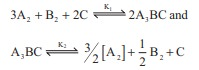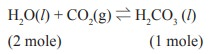# UNIT 8: Physical and Chemical Equilibrium - Online Test

Q1. If Kb and Kf for a reversible reactions are 0.8 ×10–5 and 1.6 × 10–4 respectively, the value of the equilibrium constant is,
Explaination / Solution:

Kb = 0.8 × 10–5

Kf = 1.6 × 10–4

Keq = Kf / Kb = 1.6X10-4 / 0.8X10-5 = 20

Q2.

At a given temperature and pressure, the equilibrium constant values for the equilibriaThe relation between K1 and K2 is

Explaination / Solution:Q3. The equilibrium constant for a reaction at room temperature is K1 and that at 700 K is K2. If K1 > K2, then
Explaination / Solution:

T1 = 25 + 273 = 298 K

T2 = 700 KΔHº is –ve ie., forward reaction is exothermic

Q4.

The formation of ammonia from N2(g) and H2(g) is a reversible reaction

N2(g) + 3H2(g) 2NH3(g) + Heat

What is the effect of increase of temperature on this equilibrium reaction
Explaination / Solution:

Increase in temperature, favours the endothermic reaction,

Given that formation of NH3 is exothermic ie., the reverse reaction is endothermic.

increase in temperature, shift the equilibrium to left option (c)

Q5. Solubility of carbon dioxide gas in cold water can be increased by
Explaination / Solution:increase in pressure, favours the forward reaction.

Q6. Which one of the following is incorrect statement ?
Explaination / Solution:

option (a) : wrong statement

Correct statement is, for a system at equilibrium, Q = Keq

Q7.

K1 and K2 are the equilibrium constants for the reactions respectively.What is the equilibrium constant for the reaction NO2(g) ½N2(g) + O2(g)

Explaination / Solution:Q8.

In the equilibrium,

2A(g) 2B(g) + C2(g)

the equilibrium concentrations of A, B and C2 at 400 K are 1 × 10–4 M, 2.0 × 10–3 M, 1.5 × 10–4 M respectively. The value of Kc for the equilibrium at 400 K is

Explaination / Solution:

[A] = 1 × 10–4 M ;

[B] = 2 × 10–3 M

[C] = 1.5 × 10–4 M

2A (g) 2B(g) + C2(g)Q9. An equilibrium constant of 3.2 × 10–6 for a reaction means, the equilibrium is
Explaination / Solution:

Kc = [Products] / [Reactants]

3.2 x 10-6 = [Products] / [Reactants]

KC < 10-3 ; indicates

that [Reactant] >> [Product]

option (b) is correct,largely towards reverse direction.

Q10. KC/ KP for the reaction, N2(g) + 3H2(g) 2NH3(g) is
Explaination / Solution:

for the reaction,

N2(g) + 3H2(g) 2NH3(g) ;

Δng = 2 – 4 = – 2

KP = KC (RT)–2

Kc/Kp = (RT)2